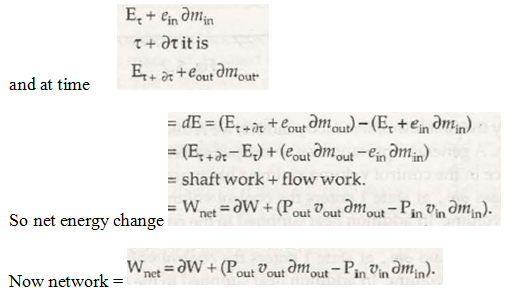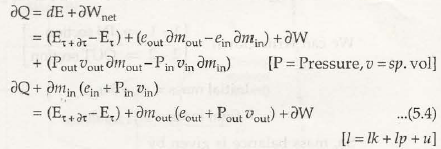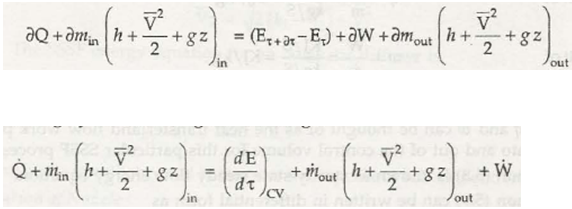When it comes to law of conservation of energy, it states:

• In closed system, you will find that total amount of energy would remain same.
• In case of closed system, the total energy would always stay constant as it can neither be created not be destroyed.
• Energy cannot be created or destroyed; it can get transformed from one to another form.

There is a possibility that the complete energy found in a system may not get transformed completely. In case of change in energy, it gets transformed to another form.

Therefore, it can be said that the law of conservation of energy is the change in energy that occurs in an object due to transformation which is equal to that of work performed on object.Conservation of energy formula

The basic formula related to the concept is:

Energy spend in one act = Energy gained in related act

An object when you drop from a height may transform its potential energy into kinetic energy. When it is expressed in mathematical term, it is said as conservation of energy equation:Mgh = ½ mv2

• m is said to be the mass of object
• v is final velocity that may happen while object falls from height h and g is said to be acceleration that occurs due to gravity

When it comes to electrical energy that is found in electric heater, it gets converted to thermal energy that may increase temperature of any substance that gets heated. The conservation of energy equation may be written as:W x t = m x s x T

• W is referred to electrical power of heater
• T is time for heating
• S is specific heat of liquid
• T is temperature of liquid

Links of Previous Main Topic:-

Links of Next Mechanical Engineering Topics:-

### Submit Your Assignment### Customer Reviews

My Homework Help
Rated 5.0 out of 5 based on 510 customer reviews at